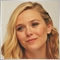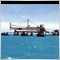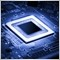• 概述
• 评论 (6)
• 评论

# ImpPower

The standard Bears Power indicator is calculated as a difference between Low price and exponential Moving Average (EMA) calculated using Close prices. The standard Bulls Power indicator is calculated as a difference between High price and EMA on Close prices.

This indicator allows:

• calculating Bears Power or Bulls Power,
• selecting method of the Moving Average: simple (SMA), exponential (EMA), smoothed (SMMA), linear weighted (LWMA), double exponential (DEMA), triple exponential (TEMA),
• calculating Moving Average on the basis of any combination of 4 basic prices: Close, Open, High, and Low.

Input parameters:

• Power - Bears or Bulls;
• Period - the period of the Moving Average;
• Moving Average mode - the averaging method: simple (Simple, SMA), exponential (Exponential, EMA), smoothed (Smoothed, SMMA), linear weighted (Linear Weight, LWMA), double exponential (Double Exponential, DEMA), triple exponential (Triple Exponential, TEMA).
• Close - weight coefficient of CLOSE price for calculation of the individual price;
• Open - weight coefficient of OPEN price for calculation of the individual price;
• High - weight coefficient of HIGH price for calculation of the individual price;
• Low - weight coefficient of LOW price for calculation of the individual price.

Example 1. Calculate the Moving Average by OPEN price: Close=0, Open=1, High=0, Low=0.
Example 2. Calculate the Moving Average by WEIGHTED price: Close=2, Open=0, High=1, Low=1.
Example 3. Calculate the Moving Average by individual price constant: Close=6, Open=7, High=1, Low=3. Price=(6*Close + 7*Open + 1*High + 3*Low)/17.424
2019.11.04 18:01

ok, help me to use16478
Nikolay Gaylis 2019.01.14 08:095669
2017.11.09 14:506157
2017.07.21 18:217173
2016.03.14 04:237826
2014.04.04 09:49